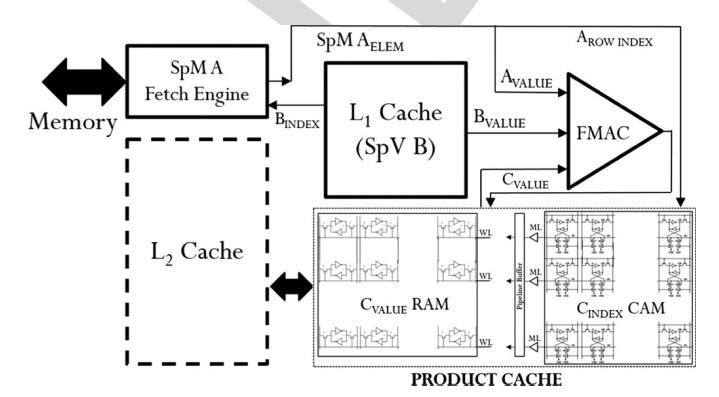•## Accelerator for Sparse Machine Learning

Sparse linear algebra is a frequent bottleneck in machine learning and data mining workloads. The efficient acceleration of sparse matrix calculations becomes even more critical when applied to big data problems. The goal is to implement an accelerator for multiplying a sparse matrix with a sparse vector. Current solutions fetch from memory all non-zero elements of the sparse matrix. The aim of this project is to implement a technique in...
Tags: